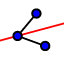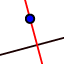# Points of Concurrency

A point of concurrency is a point where three or more lines intersect. A circumcenter is made by constructing all the perpendicular bisectors of a triangle. A very useful characteristic of a circumcenter is that it is equidistant to the verticies of a triangle. This is shown by making a circle that goes all the way around it and through all of the verticies.

 1Select tool Polygon. Create an arbitrary triangle ABC by clicking three times in the Graphics View. Close the triangle by selecting the first point A again. 2Activate tool Perpendicular Bisector. Construct the Perpendicular Bisector for two of the edges of the triangle by successively selecting the segments. Hint: You can find this tool in the Special Lines Toolbox (fourth Toolbox from the left). 3Create intersection point D of the two the line bisectors. Hint: Successively select the two line bisectors, or click directly on the intersection point. 4Construct a circle with center D through one of the vertices of triangle ABC. Hint: First, select point D, then, for example, point A. 5Select the Move tool and drag the vertices of the triangle in order to check if your construction is correct.
A reminder, a point of concurrency is a point where three or more lines intersect. An incenter is made by constructing all the anglel bisectors of a triangle. A very useful characteristic of a circumcenter is that it is equidistant to the sides of a triangle. This is shown by making a circle that goes stays inside the triangle and intersects all three in just one point each.

## Incenter Use the arrows in the bottom right to go through step by step.

 1.Select tool Polygon. Create an arbitrary triangle ABC by clicking three times in the Graphics View. Close the triangle by selecting the first point A again. 2.Activate tool Angle Bisector. Construct the Angle Bisector for each angle of the triangle. Hint: You can find this tool in the Special Lines Toolbox (fourth Toolbox from the left). 3.Create intersection point D of the line bisectors. Hint: Successively select the line bisectors, or click directly on the intersection point. 4. 5. 6.Activate tool Perpendicular Line. Construct a Perpendicular Line by selecting the Incenter made in step 3 and then a side of the triangle. Repeat this for each side of the triangle. Create the intersection of the Perpendicular Lines made in step 4 and the sides of the triangle. Construct a circle with center D through one of the intersection points made in step 5. 7.Select the Move tool and drag the vertices of the triangle in order to check if your construction is correct.